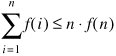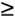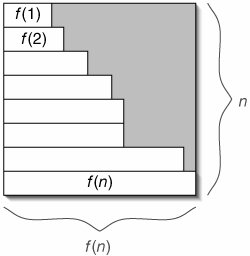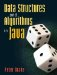# C.5. Upper Limit on Sum of a Function

We often deal with monotonically nondecreasing functions, such as running-time functions for algorithms. It is easy to state an upper limit on a sum of applications of such a function.Figure C-4 shows why this is true.

Figure C-4. Since f(n)i) for any i < n, the sum falls within an f(n) x n rectangle.### C 6 Constant FactorsData Structures and Algorithms in Java
ISBN: 0131469142
EAN: 2147483647
Year: 2004
Pages: 216
Authors: Peter Drake